Parents can use ICSE Class 9 Maths Solutions S Chand Chapter 13 Circle Ex 13(B) to provide additional support to their children.

## S Chand Class 9 ICSE Maths Solutions Chapter 13 Circle Ex 13(B)

Question 1.
Find:Solution:
(i) ∵ Angles at a point = 360°
∴ 4x – 2 + 6x + 6 + 7x – 18 = 360°
⇒ 17x – 14 = 360°
⇒ 17x = 360° + 14
⇒ 17x = 374°
⇒ x = $$\frac { 374° }{ 17 }$$ = 22°
Now, m$$\overparen{Q R}$$ = 7x – 18 = 7 x 22 – 18°
= 154°- 18° = 136°

(ii) ∵ AD is the diameter of the circle with centre O
∴ m∠AOD = 180°
∴ 10y = 180° ⇒ y = $$\frac { 180° }{ 10 }$$= 18°
Now, m∠BOC = 6y = 6 x 18 = 108°

(iii) In the figure,
$$\overparen{A C}$$ = $$\overparen{B C}$$
∴ ∠AOC = ∠BOC
∴ 8y – 8 = 6y
⇒ 8y – 6y = 8
⇒ 2y = 8
⇒ y = 4
∴ m∠AOC = 8y – 8 = 8 x 4 – 8 = 32 – 8 = 24°
∴ m∠AOC = 24°

(iv) In the given figure,
$$\odot$$ A = $$\odot$$ B
⇒ Circles with centres A and B are equal
and $$\overparen{\mathrm{CD}}=\overparen{\mathrm{EF}}$$
45 – 6x = 9x ⇒ 45 = 9x + 6x
⇒ 15x = 45 ⇒ x = $$\frac { 45 }{ 15 }$$ = 3
Now, m∠EBF = 9x = 9 x 3 = 27°
OR
If m∠CAD = 45 + 6x, then
45 + 6x = 9x ⇒ 45 = 9x – 6x
⇒ 3x = 45 ⇒ x = $$\frac { 45 }{ 15 }$$ = 15
m(∠EBF) = 9x = 9 x 15 = 135°

(v) In the given figure, a circle with centre O and QT and PS are diameters
$$m \overparen{\mathrm{PR}}=\overparen{\mathrm{PQ}}+m \overparen{\mathrm{QR}}$$
= m$$\overparen{S T}$$ + mQR
(∵ ∠POQ = ∠SOT vertically opposite angles)
= 55° + 100° = 155°
and m$$\overparen{PRT}$$ = m∠PQ + m∠QRT
= 55° + 180° = 235°(vi) In the figure, chord AB = chord CD
∴ 4y + y = y + 68°
(Angles at the centre by two equal chords are equal)
5y = y + 68° ⇒ 5y – y = 68°
⇒ 4y = 68°
⇒ y = $$\frac { 68° }{ 4 }$$ = 17°
m$$\overparen{A B}$$ = 4y + y = 5y
= 5 x 17° = 85°

Question 2.
∆PQR is inscribed in a circle. ∠P = ∠Q. Prove that PR = QR.
Solution:
∆ABC is inscribed in a circle and ∠P = ∠Q
∴ QR = PR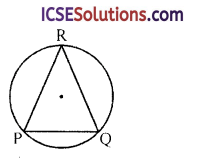∵ Equal chords subtend equal angles at the centre
∴ mPR = mQR

Question 3.
Given AB = CD. Prove that AC = DB.Solution:
In the given figure,
AB = CD
AC and BD are joined
To prove : AC = DB
∵ AB = DC
∴ m$$\overparen{A B}$$ = m$$\overparen{D C}$$
Subtracting m$$\overparen{A D}$$ from both sides
∴ m$$\overparen{A B}$$ – $$\overparen{A D}$$ = m$$\overparen{D C}$$ – m$$\overparen{A D}$$
⇒ m$$\overparen{A C}$$ = m$$\overparen{D B}$$
⇒ AC = DB
Hence proved.

Question 4.
Given AC = BD. Prove that AB = CD.
Solution:
In the figure, AC = DBTo prove : AB = DC
∵ AC = DB
∴ $$\frac { 1 }{ 2 }$$
Adding m$$\overparen{A D}$$ to both sides
$$\overparen{\mathrm{AC}}+\overparen{D B}=\overparen{A D}+\overparen{D B}$$
$$\overparen{C D}=\overparen{A B}$$
CD = AB ⇒ CD = AB
Hence AB = CD

Question 5.
In figure, X, Y are the middle points of the arcs AB, AC. Prove that AP = AQ.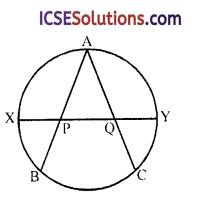Solution:
Given : in the circle,
AB and AC are two arcs.
X and Y are the midpoints of arc AB and arc AC.
XY is joined which meet AB in P and AC in Q
To prove : AP = AQ
Construction : Join AX, AY, BX and BYProof: ∵ AB = AC
∴ arc AXB = arc AYC
But X and Y are the midpoints of $$\overparen{A B}$$ and $$\overparen{A C}$$
∴ AX = XB and AY = YC
∴ ∠XAY = ∠XBA and ∠YAC = ∠YCA
∴ ∠XAB or ∠XAP = ∠YAQ
Now in ∆XAP and ∆YAQ
AY = AY (common)
∠XAP = ∠YAQ (proved)
∠AXP = ∠AYQ (proved)
∴ ∆XAP ≅ ∆YAQ (AAS axiom)
∴ AP = AQ (c.p.c.t.)
Hence proved.Question 6.
Circle O with chords AB = BC = CD = DE. Prove that AD = BE.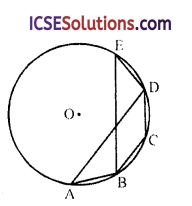Solution:
Given : In a circle with centre O, chord AB = BC = CD = DE
To prove : AD = BE
Construction : Join AO, BO, CO, DO and EO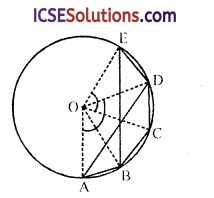Proof:
∵ AB = BC = CD = DE(given)
∴ $$\overparen{A B}+\overparen{B C}+\overparen{C D}=\overparen{A D}$$
Similarly $$\overparen{B C}+\overparen{C D}+\overparen{D E}=\overparen{B E}$$
$$\overparen{A B}+\overparen{B C}+\overparen{C D}=\overparen{B C}+\overparen{C D}+\overparen{D E}$$
⇒ $$\overparen{\mathrm{AD}}=\overparen{\mathrm{BE}}$$
∴ Central ∠AOD = central ∠BOE Now in ∆AOD and ∆BOE,
OA = OB
OD = OE (radii of the same circle)
∠AOD = ∠BOE (proved)
∴ ∆AOD = ∆BOE (SAS axiom)
Hence proved.

Question 7.
In figure, APB and CQD are two congruent arcs. Prove that AC || BD.Solution:
Given : In a circle, arc APB = arc COD
AC and BD are joined
To prove : AC = BD
Proof: ∵ Arc APB = arc CQD (given)
{Equal arcs subtends equal angles at the circumference}
But these are alternate angles
∴ AC || BD
Hence proved.

Question 8.
In figure, ABC is equilateral, P and S are midpoints of arcs AB and AC.
Prove that PQ = QR = RS.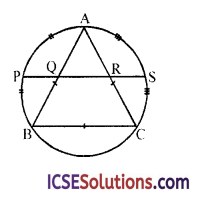Solution:
In the figure, equilateral ∆ABC is inscribed in a circle
P and S are midpoints of arcs AB and AC respectively
To prove : PQ = QR = RS
Construction : Join AP, BP, AS and CSProof: ∵ P is the midpoint of arc AB and S
is the midpoint of arc AC
∴ AP = PB and AS = SC
∠PAB = ∠PBA and ∠CAS = ∠SAC
But AB = AC (Side of equilateral triangle)
∴ ∠PAB = ∠PBA = ∠CAS = ∠SAC
Now in ∆APQ and ∆ASR,
AP = AS
∠PAQ = ∠SAR (∵ ∠PAB = ∠SAC)
and ∠APQ = ∠ASR (∵ AP = AS)
∴ ∆PAQ ≅ ∆ASR (AAS axiom)
∴ PQ = RS (c.p.c.t) … (i)
Now ∵ ∠PAQ = ∠SAR
∴ ∠PAQ + ∠QAR = ∠QAR + ∠SAR
⇒ ∠PAR = ∠SAQ
Now in ∆PAR and ∆SAQ,
AP = AS (proved)
∠PAR = ∠SAQ (proved)
∠APQ = ∠ASR (proved)
∴ ∆PAR ≅ ∆SAQ (AAS axiom)
∴ PR = QS … (ii)
Subtracting (i) and from (ii)
PR – PQ = QS – RS
QR = QR
∴ PQ = QR = RS
Hence proved.

Question 9.
Each side of a regular hexagon, inscribed in a circle subtends an angle of 60° at the centre and is equal to the radius of the circle. Prove it.
Solution:
Given : A regular hexagon ABCDEF inscribed in a circle with centre O
Join AO, BO, CO, DO, EO and FO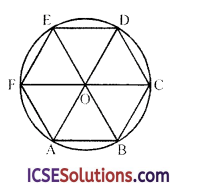To prove : Each angle of hexagon = 60°
Proof: ∵ AB = BC = CD = DE = EF = FA
(Sides of regular hexagon)
∴ $$\overparen{\mathrm{AB}}=\overparen{\mathrm{BC}}=\overparen{\mathrm{CD}}=\overparen{\mathrm{DE}}=\overparen{\mathrm{EF}}=\overparen{\mathrm{FA}}$$
∴ Each arc will subtends angle at the centre
= $$\frac { 360° }{ 6 }$$ = 60°
Hence proved.